1.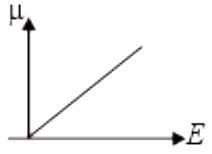2.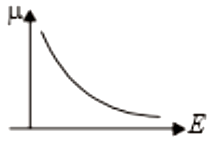3.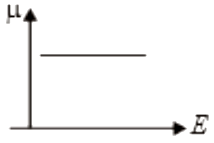4.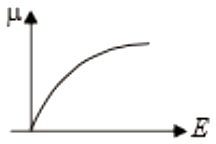Concept Questions :-

Current and current density
High Yielding Test Series + Question Bank - NEET 2020

Difficulty Level:

The equivalent resistance across AB is :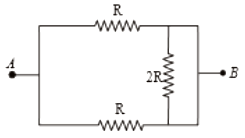1.  2R

2.  R/2

3.  4R/3

4.  3R/2

Concept Questions :-

Combination of resistors
High Yielding Test Series + Question Bank - NEET 2020

Difficulty Level:

An electric Kettle draws 4.2A at 250V. How much time it would take to boil 1kg of water at $20°$C completely to  ?

1.  320 seconds

2.  64 seconds

3.  3840 seconds

4.  960 seconds

Concept Questions :-

Heating effect of current
High Yielding Test Series + Question Bank - NEET 2020

Difficulty Level:

The equivalent resistance between points A and B in the circuit shown in the figure is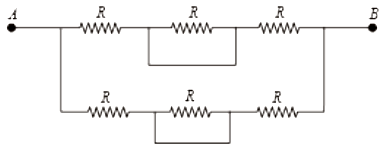1.  6R

2.  4R

3.  2R

4.  R

Concept Questions :-

Combination of resistors
High Yielding Test Series + Question Bank - NEET 2020

Difficulty Level:

A wire has resistance 24 $\Omega$. It is bent in the form of a circle. The effective resistance between the two point on the diameter of the circle is

(1) 6$\Omega$

(2) 4$\Omega$

(3) 3$\Omega$

(4) 12$\Omega$

Concept Questions :-

Combination of resistors
High Yielding Test Series + Question Bank - NEET 2020

Difficulty Level:

The drift velocity of the electrons in a current-carrying  metallic conductor is of the order of

1.

2.

3.

4.  ${10}^{-4}\mathrm{m}/\mathrm{s}$

Concept Questions :-

Current and current density
High Yielding Test Series + Question Bank - NEET 2020

Difficulty Level:

In the circuit shown in the figure, the effective resistance between A and B is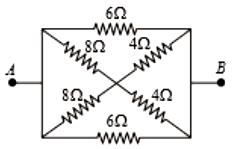1.  2$\mathrm{\Omega }$

2.  4$\mathrm{\Omega }$

3.  6$\mathrm{\Omega }$

4.  8$\mathrm{\Omega }$

Concept Questions :-

Combination of resistors
High Yielding Test Series + Question Bank - NEET 2020

Difficulty Level:

For the circuit shown in the figure, the value of R must be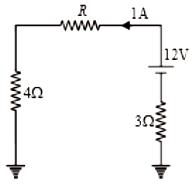1.  3$\mathrm{\Omega }$

2.  4$\mathrm{\Omega }$

3.  5$\mathrm{\Omega }$

4.  6$\mathrm{\Omega }$

Concept Questions :-

Kirchoff's voltage law
High Yielding Test Series + Question Bank - NEET 2020

Difficulty Level:

If I be the current limit of a fuse wire of length l and radius r, then select the appropriate relation

(1)

(2)

(3)

(4)

Concept Questions :-

Heating effect of current
High Yielding Test Series + Question Bank - NEET 2020

Difficulty Level:

1.  220

2.  440

3.  310

4.  550

Concept Questions :-

Derivation of Ohm's law